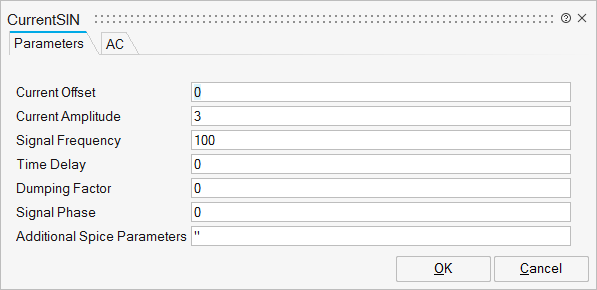# CurrentSIN

Current SourceAnalog/Basic

## Description

The CurrentSIN is a Sinusoidal current source with a constant frequency.

The peak value or amplitude value is measured from zero (if the initial DC offset value is not set) or from the DC offset value (if it is given).

## ParametersNameLabelDescriptionData TypeValid Values

sp_SIN

SIN

String

sp_I0

Current Offset

The initial DC offset current (amperes). It should be set to zero if you need a pure sinusoid.

Scalar

sp_I1

Current Amplitude

The undamped amplitude of the sinusoid, i.e. the peak value measured from zero or DC offset value (amperes)

Scalar

sp_Freq

Signal Frequency

The frequency of the sinusoid (Hertz)

Scalar

sp_Td

Time Delay

The time delay (seconds). Set this to zero for the normal sinusoid.

Scalar

sp_Theta

Damping Factor

The damping factor (Hertz). Set this to zero for the normal sinusoid. This is used to apply an exponential decay to the sinusoid.

Scalar

sp_Phase

Signal Phase

The phase advance (degrees). Set this to 90 if you need a cosine waveform.

Scalar

sp_Spice

For more details, see Additional Spice Parameters.

String

## Ports

NameTypeDescriptionIO TypeNumber

V+

implicit

Positive pin

1

V-

implicit

Negative pin

2